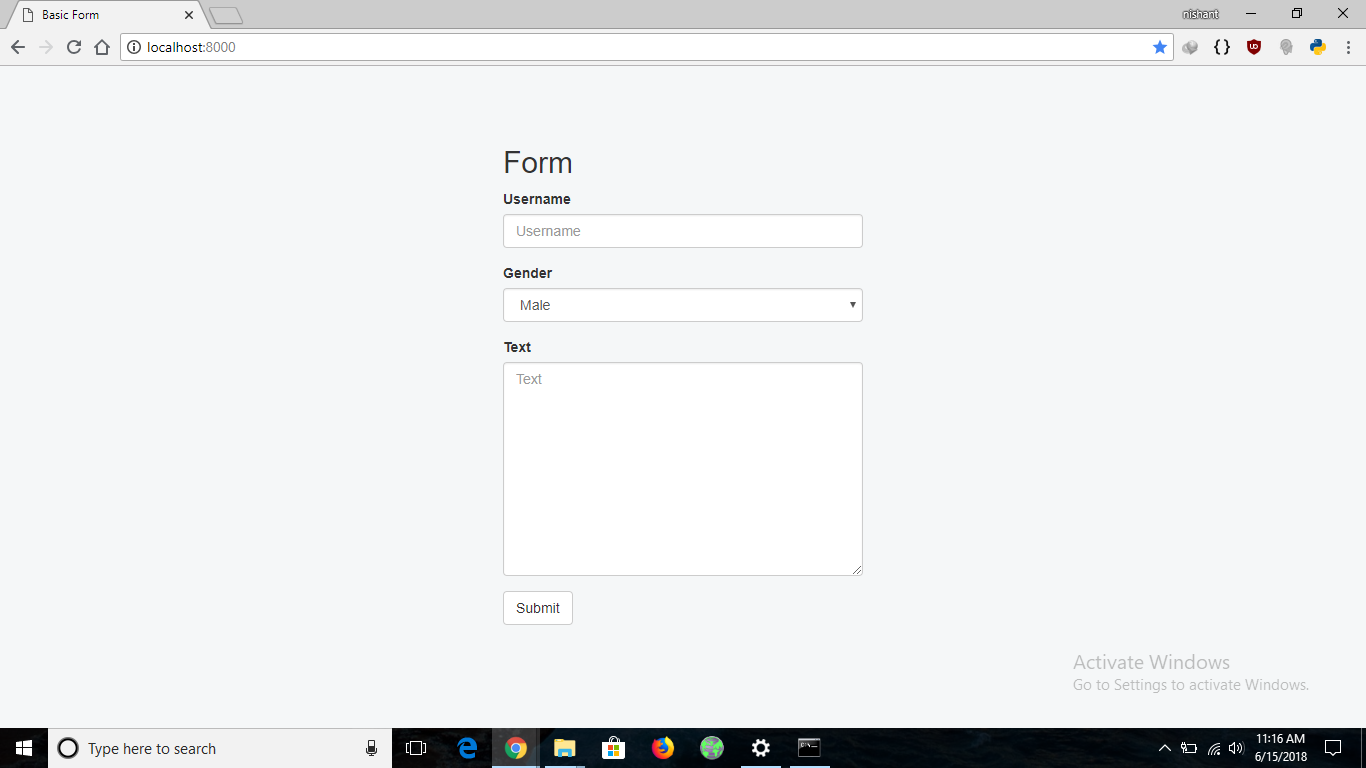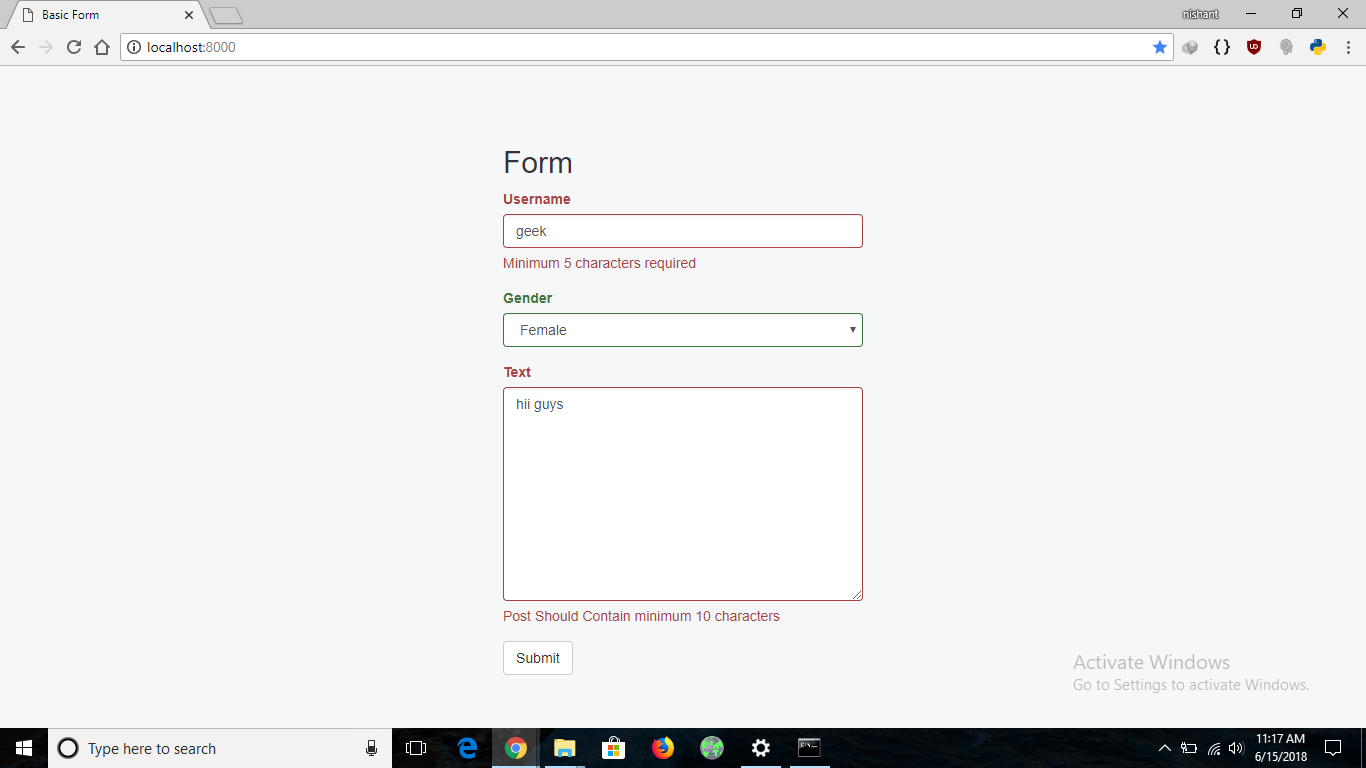Open in App
Not now

# Python | Form validation using django

• Difficulty Level : Easy
• Last Updated : 23 Jan, 2023

Prerequisites: Django Installation | Introduction to Django
Django works on an MVT pattern. So there is a need to create data models (or tables). For every table, a model class is created.
Suppose there is a form that takes Username, gender, and text as input from the user, the task is to validate the data and save it.
In django this can be done, as follows:

## Python

 `from` `django.db ``import` `models` `# model named Post``class` `Post(models.Model):``    ``Male ``=` `'M'``    ``FeMale ``=` `'F'``    ``GENDER_CHOICES ``=` `(``    ``(Male, ``'Male'``),``    ``(FeMale, ``'Female'``),``    ``)` `    ``# define a username field with bound  max length it can have``    ``username ``=` `models.CharField( max_length ``=` `20``, blank ``=` `False``,``                                 ``null ``=` `False``)``    ` `    ``# This is used to write a post``    ``text ``=` `models.TextField(blank ``=` `False``, null ``=` `False``)``    ` `    ``# Values for gender are restricted by giving choices``    ``gender ``=` `models.CharField(max_length ``=` `6``, choices ``=` `GENDER_CHOICES,``                              ``default ``=` `Male)``    ` `    ``time ``=` `models.DateTimeField(auto_now_add ``=` `True``)`

After creating the data models, the changes need to be reflected in the database to do this run the following command:

`python manage.py makemigrations`

Doing this compiles the models and if it didn’t find any errors then, it creates a file in the migration folder. Later run the command given below to finally reflect the changes saved onto the migration file onto the database.

`python manage.py migrate`

Now a form can be created. Suppose that the username length should not be less than 5 and post length should be greater than 10. Then we define the Class PostForm with the required validation rules as follows:

## Python

 `from` `django.forms ``import` `ModelForm``from` `django ``import` `forms``from` `formValidationApp.models ``import` `*` `# define the class of a form``class` `PostForm(ModelForm):``    ``class` `Meta:``        ``# write the name of models for which the form is made``        ``model ``=` `Post       ` `        ``# Custom fields``        ``fields ``=``[``"username"``, ``"gender"``, ``"text"``]` `    ``# this function will be used for the validation``    ``def` `clean(``self``):` `        ``# data from the form is fetched using super function``        ``super``(PostForm, ``self``).clean()``        ` `        ``# extract the username and text field from the data``        ``username ``=` `self``.cleaned_data.get(``'username'``)``        ``text ``=` `self``.cleaned_data.get(``'text'``)` `        ``# conditions to be met for the username length``        ``if` `len``(username) < ``5``:``            ``self``._errors[``'username'``] ``=` `self``.error_class([``                ``'Minimum 5 characters required'``])``        ``if` `len``(text) <``10``:``            ``self``._errors[``'text'``] ``=` `self``.error_class([``                ``'Post Should Contain a minimum of 10 characters'``])` `        ``# return any errors if found``        ``return` `self``.cleaned_data`

Till now, the data models and the Form class are defined. Now the focus will be on how these modules, defined above, are actually used.
First, run on localhost through this command

`python manage.py runserver`

Open http://localhost:8000/ in the browser, then it’s going to search in the urls.py file, looking for ‘ ‘ path
urls.py file is as given below:

## Python

 `from` `django.contrib ``import` `admin``from` `django.urls ``import` `path, include``from` `django.conf.urls ``import` `url``from` `django.shortcuts ``import` `HttpResponse``from` `. ``import` `views`  `urlpatterns ``=` `[``    ``path('``', views.home, name ='``index'),``]`

Basically, this associates the ‘ ‘ url with a function home which is defined in views.py file.
views.py file

## Python

 `from` `.models ``import` `Post``from` `.forms ``import` `PostForm``from` `.``import` `views``from` `django.shortcuts ``import` `HttpResponse, render, redirect`  `def` `home(request):` `    ``# check if the request is post``    ``if` `request.method ``=``=``'POST'``: ` `        ``# Pass the form data to the form class``        ``details ``=` `PostForm(request.POST)` `        ``# In the 'form' class the clean function``        ``# is defined, if all the data is correct``        ``# as per the clean function, it returns true``        ``if` `details.is_valid(): ` `            ``# Temporarily make an object to be add some``            ``# logic into the data if there is such a need``            ``# before writing to the database  ``            ``post ``=` `details.save(commit ``=` `False``)` `            ``# Finally write the changes into database``            ``post.save() ` `            ``# redirect it to some another page indicating data``            ``# was inserted successfully``            ``return` `HttpResponse(``"data submitted successfully"``)``            ` `        ``else``:``        ` `            ``# Redirect back to the same page if the data``            ``# was invalid``            ``return` `render(request, ``"home.html"``, {``'form'``:details}) ``    ``else``:` `        ``# If the request is a GET request then,``        ``# create an empty form object and``        ``# render it into the page``        ``form ``=` `PostForm(``None``)  ``        ``return` `render(request, ``'home.html'``, {``'form'``:form})`

home.html template file

## html

 `{% load bootstrap3 %}``{% bootstrap_messages %}````<``html` `lang``=``"en"``>` `<``head` `>` `    ``<``title``>Basic Form``    ` `    ``<``meta` `charset``=``"utf-8"` `/>``    ` `    ``<``meta` `name``=``"viewport"` `content``=``"width=device-width, initial-scale=1, shrink-to-fit=no"``>` `<``link` `rel``=``"stylesheet"` `href``=``"https://maxcdn.bootstrapcdn.com/bootstrap/3.3.7/css/bootstrap.min.css"``>``<``script` `src``=``"https://ajax.googleapis.com/ajax/libs/jquery/3.2.1/jquery.min.js"``>````<``script` `src``=``"https://maxcdn.bootstrapcdn.com/bootstrap/3.3.7/js/bootstrap.min.js"``>````` `<``body`  `style``=``"padding-top: 60px;background-color: #f5f7f8 !important;"``>``    ``<``div` `class``=``"container"``>``    ``<``div` `class``=``"row"``>``        ``<``div` `class``=``"col-md-4 col-md-offset-4"``>``        ``<``h2``>Form``             ``<``form` `action``=``""` `method``=``"post"``><``input` `type``=``'hidden'``/>``             ``{%csrf_token %}` `                ``{% bootstrap_form form %}````              ``<``div` `class``=``"form-group"``>``                ``<``button` `type``=``"submit"` `class``=``"btn btn-default "``>``                  ``Submit``                ``` `                  ``` `                ````        ````    ````` `` ``

Opening http://localhost:8000/ in the browser shows the following,If a form with a username of length less than 5 is submitted, it gives an error at the time of submission and similarly for the Post Text filled as well. The following image shows how the form behaves on submitting invalid form data.My Personal Notes arrow_drop_up